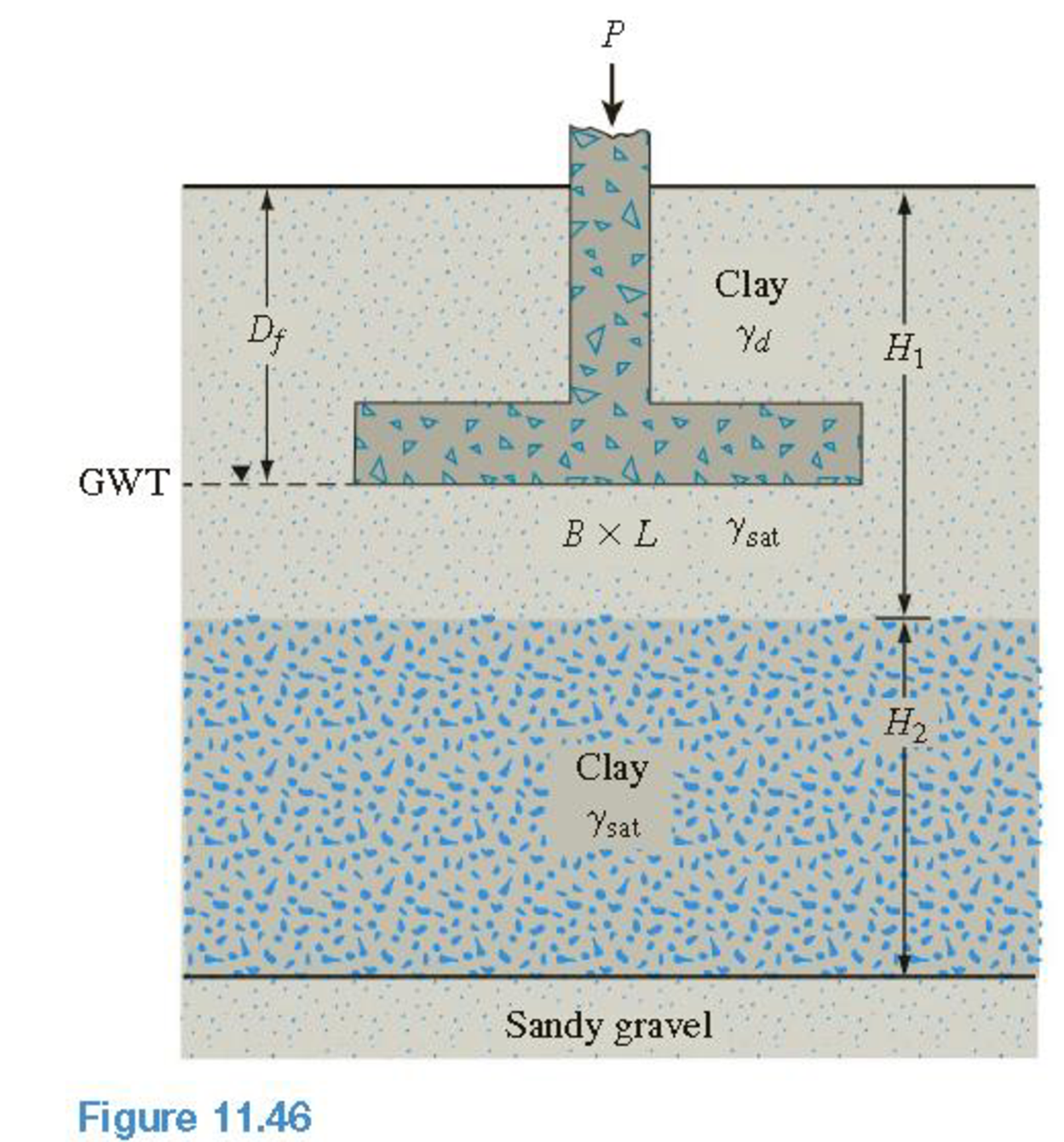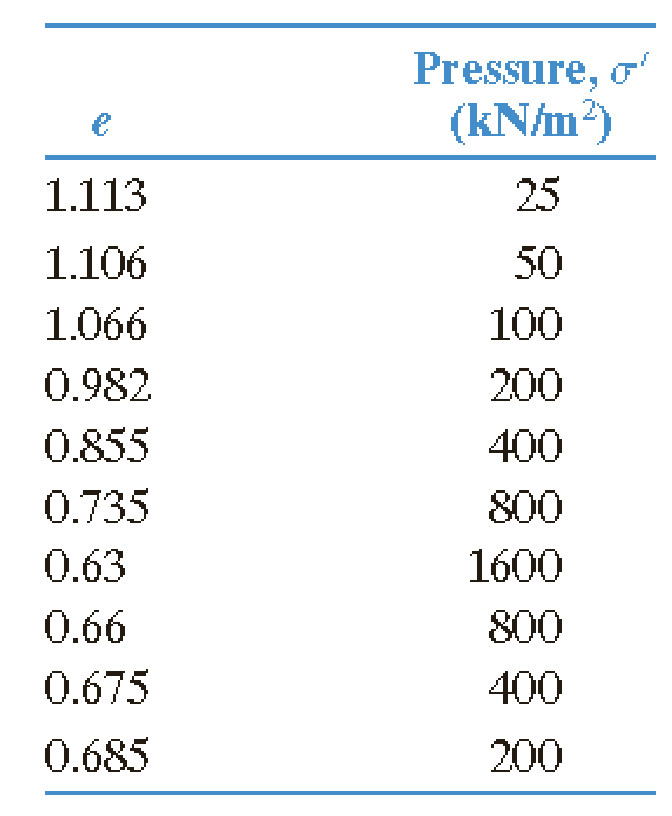Chapter 11, Problem 11.9PPrinciples of Geotechnical Enginee...

9th Edition
Braja M. Das + 1 other
ISBN: 9781305970939

Solutions

Chapter
SectionPrinciples of Geotechnical Enginee...

9th Edition
Braja M. Das + 1 other
ISBN: 9781305970939
Textbook Problem

Refer to Figure 11.46. If the consolidation properties of the clay are represented by the test results given in Problem 11.4, determine the primaryconsolidation settlement of the clay layer. Given: P = 675   kN   , B = L = 3   m , D f = 2   m , H 1 = 4   m , and H 2 = 6   m . The soil properties areSand: γd = 15 kN/m3; γsat = 18 kN/m3Clay: γsat = 18.9 kN/m3; e0 = 1.18(Use Δ σ ′ = P B L at the middle of the clay layer.)11.4 The following are the results of a consolidation test on a sample of a clayey soil.a. Plot the e-log σ′ curve. b. Using Casagrande’s method, determine the preconsolidation pressure. c. Calculate the compression index, Cc and the ratio of Cs/Cc.

To determine

Calculate the primary consolidation settlement of the clay layer.

Explanation

Given information:

The foundation load (P) is 675kN.

The length of the foundation (L) is 3m.

The breadth of the foundation (B) is 3m.

The depth of foundation (Df) is 2m.

The height of the clay layer 1 (H1) is 4m.

The height of the clay layer 2 (H2) is 6m.

The dry unit weight of sand (γd) is 15kN/m3.

The saturated unit weight of sand (γsat)sand is 18kN/m3.

The saturated unit weight of clay (γsat)clay is 18.9kN/m3.

The void ratio (e0) is 1.18.

The results (pressure and void ratio) of consolidation test on a sample of a clayey soil are given in the Table.

Calculation:

Sketch the elogσ graph using the Table values.

Show the elogσ curve as in Figure 1.

Apply the procedure to determine the preconsolidation pressure from elogσ curve as follows.

• By visual observation, set up point a, at which the elogσ plot has a minimum radius of curvature.
• Draw a horizontal line ab.
• Draw the line ac tangent at a.
• Draw the line ad, which is the bisector of the angle bac.
• Project the straight line portion gh of the elogσ plot back to the intersect line ad at f. The abscissa of point f is the preconsolidation pressure (σc).

Refer to Figure 1.

The preconsolidation pressure is represented as σc in Figure 1.

The preconsolidation pressure (σc) is 140kN/m2.

Compression index is the slope of the elogσ curve.

Refer to Figure 1.

Take the effective stress (σ1) as 400kN/m2 and (σ2) as 800kN/m2.

The void ratio (e1) is 0.855 for effective stress σ1 of 400kN/m2 and (e2) is 0.735 for effective stress (σ2) is 800kN/m2.

Calculate the compression index (CC) using the relation.

CC=e1e2log(σ2σ1)

Substitute 0.855 for e1, 0.735 for e2, 400kN/m2 for σ1, and 800kN/m2 for σ2.

CC=0.8550.735log(800400)=0.120.301=0.39

Refer to Figure 1.

Take the effective stress (σ1) as 400kN/m2 and (σ2) as 800kN/m2.

The void ratio (e1) is 0.675 for effective stress σ1 of 400kN/m2 and (e2) is 0.660 for effective stress (σ2) is 800kN/m2.

Calculate the swell index (CS) using the relation as shown below.

CS=e1e2log(σ2σ1)

Substitute 0.675 for e1, 0.660 for e2, 400kN/m2 for σ1, and 800kN/m2 for σ2

Still sussing out bartleby?

Check out a sample textbook solution.

See a sample solution

The Solution to Your Study Problems

Bartleby provides explanations to thousands of textbook problems written by our experts, many with advanced degrees!

Get Started

What are the three main types of CAD geometry styles?

Precision Machining Technology (MindTap Course List)

What is a DBMS, and what are its functions?

Database Systems: Design, Implementation, & Management

What is a schema, and how is it used?

Principles of Information Systems (MindTap Course List)

What is the job of wheel cylinder stops?

Automotive Technology: A Systems Approach (MindTap Course List)

6. How would you level DFDs?

Systems Analysis and Design (Shelly Cashman Series) (MindTap Course List)